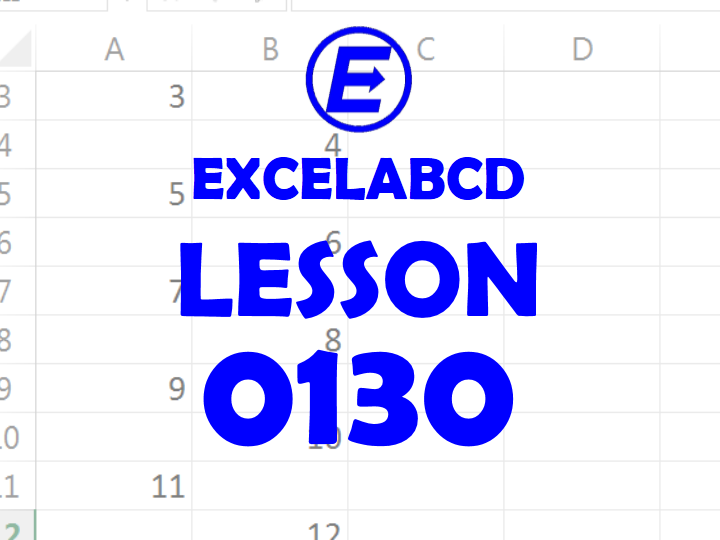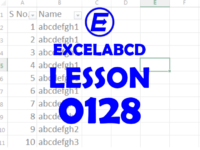## Lesson#132: How to make the function SUMPRODUCTIFIn this post I will show you the trick to make the function SUMPRODUCTIF. We have SUMPRODUCT fuction in excel. But how to make a SUMPRODUCT fuction that follows criteria like SUMIF.

Here I have made an example.

Here’s how to make the SUMPRODUCT.

Here’s the total value of all items can be found by SUMPRODUCT. But if I want to find the total value category wise then I need to modify the formula.

Here it is making SUMPRODUCT for the FRUIT category only.

And here it is making SUMPRODUCT for the VEGETABLE category only.

Instead of using this formula =SUMPRODUCT(\$F\$2:\$F\$10,G\$2:G\$10) I have used formula =SUMPRODUCT(–(\$E\$2:\$E\$10=\$E12),\$F\$2:\$F\$10,G\$2:G\$10)

What is the Double negative (“–“) does?

Double unary converts the conditions in text value to act like a numeric one and forces the rest of the function work properly considering the conditions.

We will discuss more about double unary in next posts.

Tagged with: ,

## Short Tips#0075: How to find out complete blank rows in data sheetTagged with: ,

## Lesson#131: Find out complete blank rows in data sheetIn this post I will show you a simple trick by which you can find out complete blank rows in data sheet.

I have made an arbitrary sheet to describe. I have to find out only complete blank rows in this sheet then I need to delete them.

I have added a simple formula on the very next column of the data table.

=COLUMN()-1=COUNTBLANK(A2:L2)

Now I will drag the formula up to last row of the data table. This formula will return either TRUE or FALSE.

Now I need to add filter on the header row then I will select TRUE on the column where I have put the formula.

See the result. Now I can select and delete this rows easily. In this way it is very easy to find out complete blank rows in a data sheet.

Tagged with: ,

## Lesson#130: Paste special with skip blanksHere I am having an example to show you the Paste Special with skip blanks.

Serials are incomplete in both columns. In column A even numbers are absent and in column B odd numbers are absent. If you have to paste and merge both columns then follow these steps.

Select any of the ranges and copy it then right click on the other column and click on Paste Special.

Select the Skip blanks option and click on OK.

here how it works. I hope you liked this small post.

Tagged with:

## Lesson#129: An overview on Formula AuditingHello friend! I am going to share some information about Formula Auditing in Microsoft Excel.

In the Formula ribbon you can get a group of features named Formula Auditing. It includes

• Trace Precedents
• Trace Dependents
• Remove Arrows
• Show Formulas
• Error Checking
• Evaluate Formula

Trace Precedents – Show arrows that indicate which cells affect the value of the currently selected cells.

That means you will be shown those cells from where the formula in selected cells are taking reference.

Here I have selected C1:C4 and then clicked on Trace Precedents. This shows that formulas in column C are depended on values in column A.

Trace Dependents – Show arrows that indicate which cells are affected by the value of the currently selected cells.

That means it will show those cells which are depended on the values in selected cells.

I have selected the values in range A1:A10 then clicked on Trace Dependents. It shows the C1, C2, C3, C4 cells. These four cells are depended on values in range A1:A10.

Remove Arrows – Remove all the arrows by Trace Precedents and Trace Dependents.

Show Formulas – These option shows all the formulas. Shortcut is Ctrl+`

Error Checking – Checks for common errors that occurs when using formulas.

Evaluate Formula – These feature helps you to debug a complex formula, by evaluating each part of the formula individually.

These feature can really help you when you build a complex formula.

Tagged with:

## Lesson#128: Formula to make serial no auto arranged when filteredHere I will show how to make auto arranged serial no when you filter a column. This post will be very useful to many excel users like me.

Suppose you are using a data sheet and you need filter certain portion to make print out. When you filter the data, the serial number becomes in haphazard way. To sort out this problem I have made a simple formula to auto arrange the serial no. Here is a simple example for you.

Here in the above picture I have made two example columns. First column is serial no and second one is for content or name. Added a filter on these two column.

I need to make formula to auto arrange the serial no like this.

So I have used a simple formula in place of serial no.

=IF(B2=””,””,SUBTOTAL(3,\$B\$2:\$B2))

This formula is placed in the serial no column and it will be dragged down from first cell to last cell. Now whenever you filter the sheet then the serial no will be automatically arranged starting from 1.

Tagged with: ,

## Lesson#127: Trick to make a color picker with VBAAn easy and simple trick to make a color picker will be shown here.

Here I have made a color palette by coloring different cell with different colors. This palette allows us to pick different type of color by selecting cell.

I designated a certain area where the chosen color will be shown.

Now I have inserted a button from developer tab and assigned a simple macro.

Sub Button2_Click()
Range(“O2:R8”).Interior.Color = ActiveCell.Interior.Color
End Sub

Where as Range(“O2:R8”) is the designated area for showing the picked color.

Now you have to select any color palette and click on the button to show the color in the O2;R8 area.

Here I have given the file for you.

## Lesson#126: Making a simple color tester with VBAHere I will show you how to make a simple color tester with VBA.

This color tester is having only one big button. It will take input from the user for the value of Red, Green and Blue. Then it will color the whole worksheet.

For this you have to insert a button from Developer tab and put a simple macro in it.

Sub Button1_Click()
Dim rgb1, rgb2, rgb3 As Integer
rgb1 = InputBox(“Input the value of Red between to 255”, “Red”)
rgb2 = InputBox(“Input the value of Green between to 255”, “Green”)
rgb3 = InputBox(“Input the value of Blue between to 255”, “Blue”)
Range(“A1:XFD1048576”).Interior.Color = RGB(rgb1, rgb2, rgb3)
End Sub

Now another type of color tester for you.

Here I have designated cells for input of values of Red, Green, Blue and made a data validation. Designated certain range for showing the color. Inserted a button and put this macro.

Sub Button1_Click()
Dim rgb1, rgb2, rgb3 As Integer
rgb1 = Range(“F3”)
rgb2 = Range(“F4”)
rgb3 = Range(“F5”)
Range(“G3:I5”).Interior.Color = RGB(rgb1, rgb2, rgb3)
End Sub

Here is the file for you.

Never forget to save these files in .xlsm format after using VBA.

## Lesson#125: How to use function with VBAHello we are back again with a very basic VBA learning topic. How to use function with VBA.

Here I am having an array with some random numeric values in the range A1:A10.

I want to make SUM, AVERAGE, MAX, MIN in different cells and I will use VBA for that.

So I have written code like this.

Sub calcu()
Range(“B1”).Value = “SUM”
Range(“B2”).Value = “AVERAGE”
Range(“B3”).Value = “MAX”
Range(“B4”).Value = “MIN”
Range(“C1”).Formula = “=sum(A1:A10)”
Range(“C2”).Formula = “=average(A1:A10)”
Range(“C3”).Formula = “=max(A1:A10)”
Range(“C4”).Formula = “=min(A1:A10)”
End Sub

Now it shows a result like this.

This is how to use function or formula with the use of VBA.

## Lesson#124: How to format text in excel with VBAAnother step towards learning VBA. In this post we will learn to format text in excel with VBA.

In the previous post I discussed about changing cell background color and about color codes.

So we will start in a little advance mode here. I am here taken an example of cell A1. I am going to show how to change font, text color, text size, how to change bold, italic, underline etc.

Ok then open the VBA editor by pressing Alt+F11 and then select and double click the sheet here.

#### Change background and text color

Now put code like this to change background and text color.

Sub color()
Range(“A1”).Interior.color = vbGreen
Range(“A1”).Font.color = vbRed
End Sub

This will change the background color to vbGreen or RGB(0, 255, 0) and text color into vbRed or RGB(255, 0, 0).

#### Change text size

Now I will add another line to change text size.

Sub color()
Range(“A1”).Interior.color = vbGreen
Range(“A1”).Font.color = vbRed
Range(“A1”).Font.Size = 24
End Sub

The size of text is changed in VBA like this way. I have changed into 24.

#### Change bold, italic, underline etc.

Now I will add more lines to show you how to change text to bold or italic and others.

Sub color()
Range(“A1”).Interior.color = vbGreen
Range(“A1”).Font.color = vbRed
Range(“A1”).Font.Size = 24
Range(“A1”).Font.Italic = True
Range(“A1”).Font.Bold = True
Range(“A1”).Font.Underline = True
Range(“A1”).Font.Strikethrough = True
End Sub

This has made the text bold, italic, underlined, strikethrough.

#### Change font styles.

Now I will add one more line to show how to change font style.

Sub color()
Range(“A1”).Interior.color = vbGreen
Range(“A1”).Font.color = vbRed
Range(“A1”).Font.Size = 24
Range(“A1”).Font.Italic = True
Range(“A1”).Font.Bold = True
Range(“A1”).Font.Underline = True
Range(“A1”).Font.Strikethrough = True
Range(“A1”).Font.Name = “Arial Black”
End Sub

I have changed to font style into Arial Black

#### Change cell border

This will show you how to change cell border.

Sub color()
Range(“A1”).Interior.color = vbGreen
Range(“A1”).Font.color = vbRed
Range(“A1”).Font.Size = 24
Range(“A1”).Font.Italic = True
Range(“A1”).Font.Bold = True
Range(“A1”).Font.Underline = True
Range(“A1”).Font.Strikethrough = True
Range(“A1”).Font.Name = “Arial Black”
Range(“A1”).Borders.Weight = xlThick
End Sub

I have added a thick border so I used xlThick

for thin border you have to replace with xlThin

It finally looks like this.

So we have learned about text formatting in VBA. I hope you liked this post.

Top